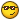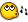# Datatype Errors

## Recommended PostsI can't seem to get the correct answer when comparing two numbers that originate from strings, but which must be compared numerically.

Here's the function where the comparison is made

```Func _Evaluate(\$pVal_1, \$psComparator, \$pVal_2, \$psDataType)
;Compares the return value from the isql against the
;threshold in the INI file .. and returns a True(1) or False(0)
;based on the comparator in the INI file

;Default RC to False
\$RC = 0

if StringUpper(\$psDataType) = "NUM" then
\$pVal_1 = Number(\$pVal_1)
\$pVal_2 = Number(\$pVal_2)
Else
\$pVal_1 = String(\$pVal_1)
\$pVal_2 = String(\$pVal_2)
Endif

_WriteLog ("Evaluating: " & \$pVal_1 & " " & \$psComparator & " " & \$pVal_2)

Select

Case StringInstr(   ":=:",         ":" & \$psComparator & ":") <> 0
If \$pVal_1 = \$pVal_2 Then \$RC = 1

Case StringInstr(   ":!=:<>:><:",  ":" & \$psComparator & ":") <> 0
If \$pVal_1 <> \$pVal_2 Then \$RC = 1

Case StringInstr(   ":>:",         ":" & \$psComparator & ":") <> 0
If \$pVal_1 > \$pVal_2 Then \$RC = 1

Case StringInstr(   ":<:",         ":" & \$psComparator & ":") <> 0
If \$pVal_1 < \$pVal_2 Then \$RC = 1

Case StringInstr(   ":>=:=>:",      ":" & \$psComparator & ":") <> 0
If \$pVal_1 >= \$pVal_2 Then \$RC = 1

Case StringInstr(   ":<=:=<:",      ":" & \$psComparator & ":") <> 0
If \$pVal_1 <= \$pVal_2 Then \$RC = 1

EndSelect

Return \$RC

EndFunc```

And here are the results

2004-03-26 (13:15:25) - Evaluating: 3 >= 25

2004-03-26 (13:15:25) - Evaluation returned TRUE: \$ynResult = 1

Any ideas how to make the comparison return False (0) for the above example?##### Share on other sites

I ran that script with:

MsgBox(0, "", _Evaluate("3", ">=", "25", "NUM"))

And it returned 0##### Share on other sitesWhen I run it like you did, I get the correct answer too .. I must have some cat by the tail - lemme go check my whole script again.

Thanks again for the prompt replies, Jon##### Share on other sites

:blush: .. ok, turns out I was NOT sending "num" in \$psDataTypeSo the world is still round after all and I haven't fallen off .. yet!Thanks, and sorry for the false alarm! :iamstupid:

## Create an account

Register a new account

• ### Recently Browsing   0 members

×

• Wiki

• Back

• #### Beta

• Git
• FAQ
×
• Create New...